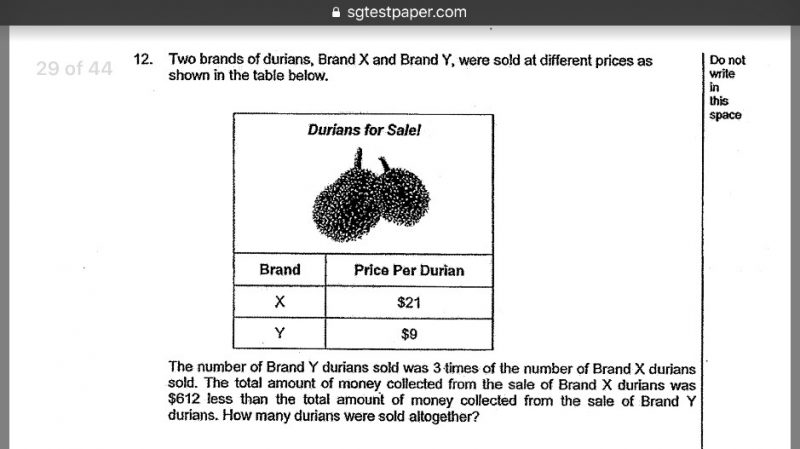# QuestionmoneyThank you in advance for a detailed working! 🙂

St Nic

Unit Price of Brand X = 21\$

Unit Price of Brand Y = 9\$

Assume:

Number of Brand X durians sold = x

Number of Brand Y durians sold = y

Equation 1 (number of Brand Y durians sold was 3 times the number of Brand X durians)

y=3x

Equation 2 (Money collected from sale of Brand X durians was \$612 less than that of Brand Y durians)

21x + 612 = 9y

21x + 612 = 9(3x)

21x + 612 = 27x

27x – 21x = 612

6x = 612

x = 102

y = 306

Total number of Durians = x + y = 408

0 Replies 0 Likes